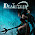## Saturday, August 25, 2012

### It's just an arbitrary rule ...

Okay, so there's an easy one for your students, right? I thought I'd try it with mine and let them argue a bit over what the answer is and why they came up with it.  When someone asks, I'll tell them that this is why we developed the arbitrary rule of PEMDAS - so that there would be no confusion, so that anyone who approached it came back with something we could all agree on.

But that's too easy, you say?

Well, following the break (Sorry to those reading in Google Reader) is a small portion of the Facebook feed. This is 100 or so of 254,542 ! According to the poster, only 30% got it right.

Shannon Bettridge Klebba Haha, just argued with my daughter about this earlier..i said 7 she said five!!
Shirley Baker I HATE MATH in ALL FORMS!
Tim McKeil Becomes 6 minus 0 plus 1. Equals 7.
Anne E Dyer This is why I only do math that I can count on my fingers. I thought the answer was 1.
Elena LadyTruthfulley JahLove damn all the time i thought x0 = 0 .....
Elena LadyTruthfulley JahLove ok 7, 5, 3,5 1 , en 4 o.O Never mind.......
Steven Lee Huff And thats why i hated math class
Claudia Raively Payne wow, john cool.....where did you learn your math??? 5X0 = 0....not 5....
Terry D Weimann ‎1....
Raymond Gagnon ‎5X0? Where did you get that? Retard!
Raymond Gagnon It's 1X0=0 & 2/2=1 so 6-0+1=7... Never add & subtract until multiplication & division is done.
Alan Michael Matthew McKee ‎6-1x0+2/2=5
1x0=0, and 2/2=1.
So,
6-+1, because the 0 no longer exists in the equation. Since its - a +, it's merely -. So, 6-1=5. Yes?
Allan Kottyan People that say BODMAS or PEMDAS doesn't apply are wrong. Not every aspect has to be there to apply the rule. There is no exponential but you still go in order. If the problem didn't include subtraction but had brackets you would use the rule even without all the components. Brackets are only needed if you want addition or subtraction applied before one of the other operations
Sarah Davis Got it right!!!! Just sayin', I'm proud of myself.=)
o
Sarah Davis ‎6-1x0+2/2
6-0+1
7.
Linda Cooley School was too long ago
Anne U Dig McLemore ‎3 and a half!!!!!!!
Anne U Dig McLemore ‎7 divided by 2?
Anne U Dig McLemore what is it? 3 n a half!
Haytham Adel ANS :1
J-Baby Cortés Then the 30 percent are the smarter ones because the ANSWER is 5 GOOGLE is wrong. What I was taught in school is to Add THEN subtract...not subtract THEN add...Its please excuse my dear aunt sally...NOT please excuse my dear sally aunt
Jake Torreano Lol u must have done the math wrong i dod it like ten tomes and still got five maube u shuld try using pemdas lol
Alex Pne Wolstenholme BODMAS - addition before subtraction. The answer is 5.
Neil Hartnell Pedmas, bodmas? When we did it, it was BIMDAS: Brackets, Indices, Multiplication, Division, Addition, Subtraction. So it appears that in different parts of the world, it's slightly different, and the operations are listed in a different order. We were taught that as long as M & D were done bore A & S, they were done in the order they appeared, so in this case, it's M, D, S, A. My calculator says 7, which is how I worked it out.
Neil Hartnell ‎*before*
Anthony Hôôch Cartwright The answer would only be 7 if the brackets were already in the equation. This is written as a straight forward sum, and therefore should be done in the order written. Answer is 1.
Cassie Mills How is it not 5? 6-0+1 is 5 under correct order of operations. Please Excuse My Dear Aunt Sally...Aunt Sally...addition and then subtraction.
Mallory Clow I will admit that I got 5 at first, but then reading these comments - I had forgotten that once you get the multiplication/division out of the way you go left to right. lol. I remembered BEDMAS, but I forgot that 1 simple rule.
Nick A Partida Regardless of brackets, multiplication and division should be worked first. Then I would agree, work it in the order its in. 6-0+1=7. This is how it was taught in school, and the person posting says it's the right answer. So does a calculator if you give it a try. Why is it this so difficult for people?
Nick A Partida Found this on mathisfun website. This is exactly how I was taught in school.

Parentheses first

E

Exponents (ie Powers and Square Roots, etc.)

MD

Multiplication and Division (left-to-right)

AS

Addition and Subtraction (left-to-right)

Michelle Ferguson Wade For those insisting the answer is not 7, go ahead and plug it into a calculator. You'll see that 7 is correct. PEMDAS is the guide. You have to remember the rule of left to right when you are left with only subtraction/addition.
Peter Jones http://en.wikipedia.org/wiki/Order_of_operation

Multiply and divide before adding and subtracting. You don't need the brackets to install that order. Everyone who insists otherwise is an idiot from this moment forward.

The true idiot award here, though, goes to J Baby Cortes, who got to the 6-0+1 and then decided the answer was 5. It isn't; addition and subtraction are done simultaneously, because you're summing numbers. The question could be rephrased as "what is the sum of 6, negative 0, and 1." The answer is 7. If you disagree, look at this post and figure out why you are the stupid one.
In mathematics and computer programming, the order of operations (sometimes call...See More
Amina Winchester Wahid God maths gives me a headAche..
Lindsay Chapman Addition and subtraction are on equal terms with each other!!

You go left to right if there is both an addition and subtraction!

Take a number out of the original equation (6=a) and put 5 in as the answer:

a-(1x0)+(2/2)=5
a-0+1=5
a=5-1
a=4

Shouldn't a=6??? Therefore 5 is wrong!

a-(1x0)+(2/2)=7
a-0+1=7
a=7-1
a=6 which is correct

This proves algebraically that 7 is correct.

Denise Siggens what was the answer?
Jj Bartrom It seems as though the answer depends on which way you look at the problem. But we can't have this kind of flexibility in mathematics; math won't work if you can't be sure of the answer, or if the exact same problem can calculate to two or more different answers. To eliminate this confusion, we have some rules of precedence, established at least as far back as the 1500s, called the "order of operations". The "operations" are addition, subtraction, multiplication, division, exponentiation, and grouping; the "order" of these operations states which operations take precedence (are taken care of) before which other operations.
Alex Hannam The people say that the add and subtract are equal and are done left to right, bam cleared it up for me... then Lindsay Chapman's thing should do it for the rest of the haters.Addition and subtraction are on equal terms with each other!!

You go left to right if there is both an addition and subtraction!

Take a number out of the original equation (6=a) and put 5 in as the answer:

a-(1x0)+(2/2)=5
a-0+1=5
a=5-1
a=4

Shouldn't a=6??? Therefore 5 is wrong!

a-(1x0)+(2/2)=7
a-0+1=7
a=7-1
a=6 which is correct

This proves algebraically that 7 is correct.
Natalie Rogers ‎:)

P
E
MD
AS

When I teach PEMDAS to my students, I make them write it like I have above. This helps them remember that you resolve multiplication and division in the same step, as they occur from left to right. The same holds true for addition and subtraction - they must be resolved as they appear from left to right. So if you have only addition and subtraction remaining - start on the left and solve each part as you go. For the above problem it means that you will solve 6-0 before adding 1.
John Tucker UGH..Kris Baldoza Yes YOU do need to go back to elementary. read the comments to see if you can figure out why the answer is 7
Chris Forgione ‎7 PEMDAS or as I was taught it, Please Excuse My Dear Aunt Sally
Geoffrey Taylor I was in algebra class yesterday and we had a question that was to test our oder of operations class very similar to this. Using PEMDAS you would do the Multiplication /Division parts FIRST. If the problem has both so you then go left to right for those parts then you go on to the Addition/subtraction parts, which once again you do left to right. So 6-1x0+2/2=7 It ls done in this order: 6-(1x0)+(2/2)= 6-(0)+(1)=7.

Yes we had a bit of discussion to clear that up for those of us that were a bit slower than others in remembering that order of operations.
Lynn Breaux The instructions say answer IT, so IT should bet he correct answer. The math, of course, comes out to 7.
Matt Rizzo multiply by all by zero = 0. 0 divide all by 2 = 0, 6+2 = 8, minus 1 = 7 (yay seven!)
Kathi Schaff Sears The answer is 7 but people please can't we just get along. The reason so many people hate math is because those that love it are so mean to those that don't get it yet. I love math because of a great 6th grade teacher that made it fun. Please remember we should all be kind to each other.
Franklin Sampley Plug it into a calculator? My calculator says 1 - so unless there's some brackets - I'll stick to one. :D
Karen Rizzo Marsh Seven - but I don't know if it is a debit or a credit?
Gary Spencer Pemdas on 6 (-1x0) (+2/2) ~~ Mult: 1x0 = 0;

tricked me!.. -0 is just 0, so no more subtraction :P
Sherman Dippel I can see how those folks that answered 3.5 got there, and wondering if any of them know what you get when you multiply anything by zero..? Not that alone will get you the right answer. If you amend that process, you'd end up with 1, which is still wrong. You have to do the multiplication and division first, THEN go left to right: 6 - (0) + (1) = 7
Marc James they are always 1
Jessica Krucek Yeah, here's how it broke down; no brackets or parenthesis. No exponents. Next is multiplication and division (same step, left to right) so 6-(0)+(1). Addition and subtraction (go left to right) leads to none from six, plus one. Seven
Joanne Coombe Woo hoo I'm smart lol
Sammie Walton ‎6-1x0+2/2
P. And E. Are no used, so start with M.
6 - (1x0) + 2/2
6 - 0 + 2\2.
Next comes D.
6 - 0 + (2/2)
6 - 0 + 1
This is where you go wrong. Pedmas states A( addition) before S (subtraction).
So, do as pedmas says.
6 - (1 + 0)
6 - 1
Now lastly, S for subtration.
6-1 = 5.
Sammie Walton ‎(pemdas and pedmas are interchangeable according to the internet o.o)
Bruce Luper I've been out of school too long
James Swann No Parenthesis, so BODMAS/PEMDAS (Whatever you know it as) does NOT apply so it's just left to right.
6 - 1 = 5
5 x 0 = 5
5 + 2 = 7
7 divided by 2 = 3.5
Paul Anthony Baldovin To james swann -- in what universe is 5x0 equal to 5?? You sir are a moron, please dont ever comment on any math related questions again
James Swann You have 5; you times it by 0 and you STILL have five, since you STARTED with five and aren't adding, subtracting, dividing or taking anything from it; that's how.
Deana Price ‎5x0 is always ZERO
Paul Anthony Baldovin ANYTHING TIMES ZERO IS ZERO YOU MORON!! If you are multiplying by ZERO, that means you dont have anything to begin with! Wow the stupidity on facebook.. 5 + 0 = 5, 5 x 0 = 0.. Wow you are an idiot..
Paul Anthony Baldovin If i have nothing of something, and I hand nothing to 5 people.. We all have nothing.. That is essentially what 5 x 0 is = nothing! Lol
Dakota Cooper PEMDAS ALWAYS applies whether or not there are parenthesis or not. The answer is 7.
Fred Newton ‎35% got this right? I have stopped smiling.
Jen Kerchner Pyzowski Remember the rule of Please Excuse My Dear Aunt Sally? Parenthesis, Exponents, Multiplication, Division, Addition, Subtraction. Order of operations. Wish I could say I remembered this on my own,but I have kids who needed to know this and I had COMPLETELY forgotten about it until they needed to know... Oh well, I'm certainly far from perfect. :) But I did get the answer. Thank you elementary school math!
Rusty Ellison Sorry James Swan but 5x0=0. Anything times 0 ='s 0 therefor the answer is 1. There are no parentesis therefore the problum is don in order. so lets give it a shot. 6-1X0+2/2=? 6-1=5 5x0=0
Rusty Ellison ‎0+2=2........2/2=1
Rusty Ellison ‎1. I did it on the calculator and also came up with one
Rusty Ellison Here is a riddle: 3 men go to get a motel room. The ask the clerk, how much is the room? The clerk replys, \$30.00 so the three men spit it 3 ways, each putting up \$10.00. They get the key and retire to their room. A few minutes later the hotel manager comes in a realizes that the tree men were overcharged for the room by \$5.00 so he hand the clerk 5 singles to take back to the three men. Instead of taking the three men the five singles, he pockits 2 and gives them each back one dollar.(here's were it gets tricky) The three, according to the math, paid \$27.00 for the room instead \$30.00. So, \$27.00 + the \$2.00 that the clerk pockited only equals \$29.00. WHAT HAPPENED TO THE OTHER DOLLAR?
Todd Krost ‎6-1x0+2/2= 6-0+2/2 6-0+1 6+1 7
Luke Overthere Conway if u dont know, its 5
Aaron Moyer That riddle makes no sense. The guys should only have paid 25\$ for the room after their mistake. He pocketed two dollars and gave s buck to each guy. That equals the five bucks. No riddle. :)
Sarah Suenkel I got 7. I took 1x0=0, then too 6-0=6 so the left side answer is 6 then I took 2/2=1, so the right side answer is 1, then I added the left side 6 with the right side 1 and got the answer 7 this is too easy do you have a harder one?
Tanja van den Bergh When solving 6-1*0+2/2 you have to follow the rules of PEMDAS, stating a problem/equation has to be solved in the right order (Parentheses Exponents Multiplication Division Addition Subtraction). In this case, we start with solving the multiplication and division. So to solve 6-1*0+2/2 you first have to solve 1*0, which is 0. Then solve 6-0 = 6. Then we solve 2/2 which is 1, amd 6+1 is 7.
Marilyn Barker You're all mad!!
Sage Jackson They are just mad cuz they got it wrong..regardless its simple math..
6-1x0+2/2=?
1x0=0
2/2=1 so now we have
6-0+1
Someone earlier was trying to drop the 0. You can, but you will have to drop the negative in it too. Cause the equation is +6, -0, +1. =+7. Not 5.. not 1.. and sure as who the hell came up with 3.5
Melissa Fei Lowe how the fuck do you get 7? P.E.M.D.A.S. would mean multiplying the -1 and 0 wiping them out. 2/2 = 1. then 1+6= 7. BOOM.
Susan Lapane ‎3.5.I mean.
Daniel Barber The correct answer is 7. In the order of operations, relying solely on PEMDA is misleading. Multiplication and division share the same "weight" as do addition and subtraction. 6-(1x0)+(2/2)=6-0+1=7 not 5. Just as 10-3+2=9 rather than 5. Evaluate left to right for operators that share the same weight. Additionally, when doing this on a calculator it is necessary to understand how your particular calculator is doing the evaluation. To quote Wikipedia again, "The non-scientific calculator expects two operands and an operator. When the next operator is pressed, the expression is immediately evaluated and the answer becomes the left hand of the next operator. Advanced calculators allow entry of the whole expression, grouped as necessary, and evaluates only when the user uses the equals sign." https://en.wikipedia.org/wiki/Order_of_operations
en.wikipedia.org
In mathematics and computer programming, the order of operations (sometimes call...See More
Ange Taylor Brackets = there is none
Exponents = there is none
Division = 2 divided by 2 is 1 (at 1)
Multiplication = 1 multiplied by 0 is 0 (still at 1)
Addition = 0 add 2 is 2 (at 3)
Subtraction = 6 subtract 1 is 5 (at 8)

BEDMAS, the answer is 8
Charmaine Brick Ange you may need to check you're 0 times tables. I was always taught 0 multiplied by any number was 0.
Jeremy Danes Lol at Ange, you group your Division/Multiplication and Subtraction/Multiplication it doesn't entirely matter when you do them. You have to reference from left to right, you referenced from right to left. So my point is, you can divide 2 and 2 first but you have to put that number back into the equation and do the problem with your addition and subtraction. So after the BEDMA in your method, the resulting equation is 6-0+1 resulting in 7. Blouses.
Erin Lamothe BEDMAS..brackets, equations, division, multiplication, addition and subtraction.. no brackets or equations..next step would be division 2/2 which equals 1..then multiplication 1x0 which equals 0, then addition 0+1 which equals 1, then subtraction 6-1 which equals 5..the answer has to be 5. Guess it's a matter of opinion more then intelligence.
Randy Herrin Screw math! I was Better at speling!
Mike Miller ‎1 IS THE ANSWER
Mike Miller ‎(6-1X0) = 0 (2/2) = 1 ( 0 ) + ( 1 ) = 1
Betty Green Doesn't there have to be only ONE correct answer to this?
Jim Mayfield Nope. Multiply and divide first: 6-0+1=7.

1.6-(1x0)+2/2 = 6-0+(2/2) = 6-(0+1) = 6-1 =5

1.6-0+1 is not equal to 6-(0+1)

6-0+1 is the same as adding...
+6
-0
+1
--
7

Try any of these calculators...enter 6-1*0+2/2...
http://www.wolframalpha.com/
http://www.encalc.com/
http://web2.0calc.com/

2.6-1x0+2/2
a-1x0+2/2=5
a-(1x0)+(2/2)=5
a-0+1=5
a-1=5
a=6 correct

6-1x0+2/2
a-1x0+2/2=7
a-(1x0)+(2/2)=7
a-0+1=7
a-1=7
a=8 wrong

1.Picking up at this point...
a-0+1=7
a-0+1-1=7-1 (subtraction 1 from both sides)
a-0=6
a=6

So 6-1x0+2/2 = 7

3.You can't make the leap that 6-0+1 = 6-1.
If it was stated as 6-(0+1), then the answer is 5.
But without parentheses, 6-0+1 should be solved left to right, as addition and subtraction have no precedence over the other.

6-0+1 = 6+1 = 7

4.Some correctly restate the problem as 6-0+1, but fail to solve it correctly. Since addition and subtraction have no precedence over the other in the order of operations, many think of it as solving left to right...

6-0+1 = 6+1 = 7

It can also be considered as this addition problem...

+6
-0
+1
--
7

5.You're all getting WAY too upset. The answer is 5. Multiplication DOES come first, but it doesn't mean you take the two numbers AROUND the 'x' symbol and multiply them (as that would be arbitrary and make no sense), it means you SEPARATE the equation out from that point. That's why everyone's 'arguing,' because they don't know what the Order of Operations is dictating. When this is done correctly, the equation is reduced to:

1. (6-1) x (0+2/2)

Then, from there, we do division. It doesn't matter that the multiplication isn't DONE yet, because as you can tell, we haven't reduced the equation fully, according to how PEMDAS actually works:

2. (6-1) x (0+1)

Now, it's easy. (5 x 1) This is why the answer is five, and only five.

1.But PEDMAS gives multiplication and division equal weight, yet you left the multiplication until last but did the division first. You are simply wrong.

6-1x0+2/2

6-(1x0)+(2/2)

6-0+1

7

THAT is pedmas

6.To the anonymous remark on Sept. 20:

Try all of these online calculators - you will get the result of 7 each time...

http://www.wolframalpha.com/
http://www.encalc.com/
http://web2.0calc.com/

For each one, enter the expression as...

6-1*0+2/2

Or use Excel...
=6-1*0+2/2

7.Order of Ops says addition before subtraction. So its 5. All of you saying 7 are subtracting before you add. Order of Ops is a crock! Any handheld calculator says its 1.

1.Nope, Order of Ops give addition and subtraction the same importance and they are done left to right.
(PE)(DM)(AS)

8.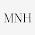BODMAS
6-1*0+2/2
6-1*0+(2/2)
6-(1*0)+1
6-0+1
7

9.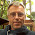Answering anything other than 7 is just embarrassing (see why:Why 6-1*0+2/2=7). It just makes me wonder how people finish their education if they fail to learn basics in 5th or 6th grade.

10.I never understood this, while PEMDAS is good to know...I have never used it in real life. 22 years in the work force and have yet to use this

11.If you start out with 6 chickens and add none you have 6. Now if I multiply by 0 hmmm I'd still have 6 but somehow I can't use them any more cuz the math works that way. So I'm guessin some nice person gave me 2 to use,I'd divide them in two and put em in a single coop so I'm gonna say I got 1 coop of chickens. Trouble I have is these 6 other chickens here. I'm gonna have to build another coop. Huh,math is weird.

12.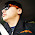13.I keep getting 4! Lol! I get the 7 and 1, but doing it in order I still keep getting 4 doing it 2 separate ways

14.I keep getting 4.. I also get the 7-1, but trying it in two forms I still get 4?...

15.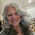16.Why do people keep entering the incorrect answer? When someone has explained that it is 7, please read WHY this is 7. As 7 actually is the one and only correct answer to this. This is not a debate. Not a discussion. It's math. According to the rules (and logic) the answer is 7. NOTHING ELSE!

17.